# Texas Go Math Grade 4 Lesson 15.5 Answer Key Mixed Measures

Refer to our Texas Go Math Grade 4 Answer Key Pdf to score good marks in the exams. Test yourself by practicing the problems from Texas Go Math Grade 4 Lesson 15.5 Answer Key Mixed Measures.

## Texas Go Math Grade 4 Lesson 15.5 Answer Key Mixed Measures

Essential Question

How can you solve problems involving mixed measures?
By converting them to single measures and then solve

Unlock the Problem

Herman is building a picnic table for a new campground. The picnic table is 5 feet 10 inches long. How long is the picnic table in inches?
70 inches is the picnic table

Is the mixed measure greater than or less than 6 feet?
1 foot = 12 inches
6 x 12 = 72 inches.
5 feet 10 inches long.
converting to inches
5 x 12 = 60
60 + 10 = 70
72 > 70
the mixed measure is less than 6 feet

How many inches are in 1 foot?
1 foot = 12 inches

Change a mixed measure.

Think of 5 feet 10 inches as 5 feet + 10 inches.
Write feet as inches.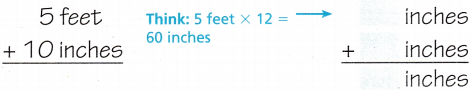So, the picnic table is ________ inches long.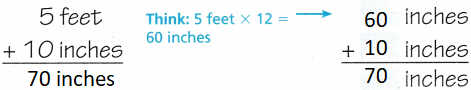Explanation:
So, the picnic table is 70 inches long.

Herman built a deck behind his house in 2 days. The first day he worked he made the deck 12 feet 6 inches wide. The second day he worked he made the deck 8 feet 4 inches wider. How wide is the deck that Herman built?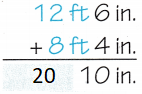So, the deck Herman built was _______________ wide.
So, the deck Herman built was 20 feet 10 inch wide.

What if Herman added an extra 2 inches on the deck width? How wide would the deck be then? Explain.
21 feet
1 foot = 12 inch
10 + 2 = 12
so, 20 feet 10 inch + 2 inch = 21 feet.

Math Talk

Mathematical Processes
How is adding mixed measures similar to adding tens and ones? it different? Explain.
Yes

Example 2 Subtract mixed measures.
Alicia is building a fence around the picnic area. She has a pole that is 6 feet 6 inches long. She cuts off 1 foot 7 inches from one end. How long is the pole now?

ERROR Alert: Be sure to check that you are regrouping correctly. There are 12 inches in 1 foot.

STEP 1 Subtract the inches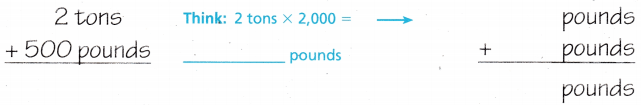STEP 2 Subtract the feet.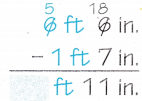So, the pole is now _____________ long.
So, the pole is now 4 feet 11 inch long.

Try This! Subtract.

3 pounds 5 ounces – 1 pound 2 ounces
3 pounds 5 ounces – 1 pound 2 ounces =
4 pounds 3 ounces

4 gallons 7 cups – 1 gallon 12 cups
1 gallon = 16 cups
4 gallons 7 cups
converted as 3 gallons 23 cups
3 gallons 23 cups – 1 gallon 12 cups = 2 gallon 11 cups

Share and Show

Question 1.
A truck is carrying 2 tons 500 pounds of steel. How many pounds of steel is the truck carrying?
Think of 2 tons 500 pounds as 2 tons + 500 pounds. Write tons as pounds.So, the truck is carrying ____________ pounds of steel.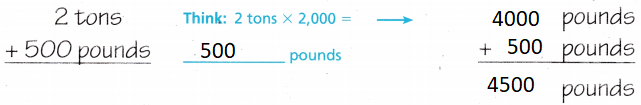Explanation:
So, the truck is carrying 4500 pounds of steel.

Rewrite each measure in the given unit.

Question 2.
1 yard 2 feet
____________ feet
1 yard = 3 feet
3 + 2 = 5
Explanation:
5 feet

Question 3.
3 pints 1 cup
____________ cups
7 cups
Explanation:
1 pint = 2 cups
3 x 2 = 6
6 + 1 = 7

Question 4.
3 weeks 1 day
____________ days
22 days
Explanation:
1 week = 7 days
3 x 7 = 21
21 + 1 = 22

Question 5.Explanation:
1 pound = 16 ounces

Question 6.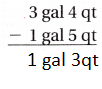Explanation:
1 gallon = 4 quarts

Question 7.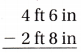Explanation:
1 feet = 12 inches

Math Talk

Mathematical Processes
How do you know when you need to regroup to subtract? Explain.
If there is no sufficient amount to subtract
we have to regroup.

Problem Solving

Question 8.H.O.T. Justify Jackson has a rope 1 foot 8 inches long. He cuts it into 4 equal pieces. How many inches long is each piece?
5 inches long is each piece
Explanation:
1 foot = 12 inches
12 + 8 = 20 inches
20 ÷ 4 = 5 inches

Question 9.
H.O.T. Sense or Nonsense? Sam and Dave each solve the problem at the right. Sam says the sum is 4 feet 18 inches. Dave says the sum is 5 feet 6 inches. Whose answer makes sense? Whose answer is nonsense? Explain.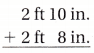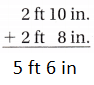Explanation:
2 ft + 2 ft = 4 fts
10 in + 8 in = 18 inches = i ft 6 inches   since 12 inches equals to 1 ft
So 4 fts + 1ft 6 inches= 5 ft 6 in.

Question 10.
H.O.T. Multi-Step Don has 5 pieces of pipe. Each piece is 3 feet 6 inches long. If Don joins the pieces end to end to make one long pipe, how long will the new pipe be? Explain.
Explanation:

3 feet 6 inches + 3 feet 6 inches+3 feet 6 inches+3 feet 6 inches+3 feet 6 inches =15 fts 30 inches
1ft =12 inches
convert 30 inches to fts is 12 inches + 12 inches + 6 inches = 1ft+ 1 ft+6 inches= 2 ft 6 inches
15fts 30 in= 15fts + 2 fts 6 inches= 17fts 6 inches

Question 11.
Mark had a dog that weighed 21 pounds 8 ounces. The dog lost 1 pound 14 ounces. How much does the dog weigh now?
(A) 20 pounds 4 ounces
(B) 20 pounds 10 ounces
(C) 19 pounds 10 ounces
(D) 19 pounds 4 ounces
Explanation:
Mark had a dog that weighed 21 pounds 8 ounces
From 21 pounds 8 ounces convert 2 pounds to ounces. So it is 19 pounds 40 ounces.
The dog lost 1 pound 14 ounces. So dog lost 30 ounces, since 1 pound equals to 16 ounces.
19 pounds 40 ounces- 30 ounces= 19 pounds 10 ounces
Question 12.
Linda is making a costume. She needs 3 feet 9 inches of purple fabric and 4 feet 7 inches of orange fabric. How much fabric does Linda need to make the costume?
(A) 8 feet 16 inches
(B) 7 feet 4 inches
(C) 8 feet 4 inches
(D) 1 foot 4 inches
Explanation:
3 feet 9 inches of purple fabric + 4 feet 7 inches of orange fabric = 7 feet 16 inches
1 feet = 12 inches
convert 16 inches to feet= 1 feet 4 inches.
7 feet 16 inches = 7 feet + 1 feet 4 inches. = 8 feet 4 inches

Question 13.
Multi-Step Tara makes orange smoothies for a party. She combines 2 gallons 3 quarts of milk with 1 gallon 2 quarts of orange juice. Party guests drink 2 gallons 3 quarts of the smoothies. How much is left of the orange smoothie?
(A) 4 gallons 1 quart
(B) 1 gallon 2 quarts
(C) 2 gallons 2 quarts
(D) 1 gallon 1 quart
Explanation:
Total Smoothie is 2 gallons 3 quarts of milk + 1 gallon 2 quarts = 3 gallons 5 quarts
Party guests drink 2 gallons 3 quarts of the smoothies
3 gallons 5 quarts – 2 gallons 3 quarts = 1 gallon 2 quarts

Question 14.
Maya’s cat weighed 7 pounds 2 ounces last year. The cat gained 1 pound 8 ounces this year. What is the weight of Maya’s cat now?
(A) 5 pounds 10 ounces
(C) 8 pounds 10 ounces
(C) 8 pounds 2 ounces
(D) 9 pounds
Explanation:
1 pound = 16 ounces
convert 2 pounds to ounces  from 7 pounds 2 ounces = 5 pounds + 2 pounds 2 ounces = 5 pounds 34 ounces
1 pound 8 ounces= 24 ounces
5 pounds 34 ounces – 24 ounces = 5 pounds 10 ounces

### Texas Go Math Grade 4 Lesson 15.5 Homework and Practice Answer Key

Mixed Measures

Rewrite each measurement in the given unit.

Question 1.
1 pound 8 ounces
___________ ounces
Explanation:
1 pound equals to 16 ounces
16 + 8 = 24 ounces

Question 2.
3 feet 1 inch
___________ inches
Explanation:
1 feet = 12 inches
3 feet = 36 inches
3 feet 1 inch = 37 inches

Question 3.
2 gallons 3 quarts
___________ quarts
Explanation:
1 gallon = 4 quarts
2 gallons 3 quarts = 4 quarts +4 quarts+ 3 quarts = 11 quarts

Rewrite each measurement in the given unit.

Question 4.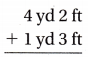Explanation:
1yd = 3ft
4yd 2 ft + 1 yd 3 ft = 5 yd 5 ft=6 yd 2ft

Question 5.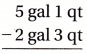Explanation:
1 gal = 4 qts
5 gal 1 qt = 4 gal 5 qt
4 gal 5 qt – 2 gal 3 qts = 2 gal 2 qt

Question 6.Explanation:

1 lb = 16oz
2 lb 10oz + 3 lb 12oz= 5lb 22oz=5lb + 16oz + 6 oz = 6lb 6 oz

Problem Solving

Question 7.
Clayton filled 5 containers with water. Each container holds 2 gallons and 2 quarts. How many quarts of water does Clayton have? Explain.
Explanation:
5 X 2 gallons and 2 quarts = 10 gallons 10 quarts
1 gallons = 4 quarts
10 gallons 10 quarts  = 10 gallons + 4 quarts  + 4 quarts +2 quarts = 12 gallons 2 quarts

Question 8.
Michael bought 2 pounds 12 ounces of cashews and 3 pounds 6 ounces of almonds. How many more ounces of almonds did Michael buy? Explain.
Explanation:
1 pound = 16 ounces
3 pounds 6 ounces – 2 pounds 12 ounces = 2 pounds 22 ounces – 2 pounds 12 ounces =10 ounces

Lesson Check

Question 9.
Multi-Step A snake is 2 feet 4 inches long. How many inches long is the snake?
(A) 28 inches
(B) 76 inches
(C) 24 inches
(D) 42 inches
Explanation:
1 feet = 12 inches
2 feet 4 inches = 12 inches+12 inches+ 4 inches= 28 inches

Question 10.
Mike has a board that is 38 inches long. How many feet and inches is the board?
(A) 1 foot 11 inches
(B) 2 feet 8 inches
(C) 3 feet 1 inch
(D) 3 feet 2 inches
Explanation:
1 feet = 12 inches
12 inches+12 inches+12 inches+ 2 inches = 1ft + 1ft + 1ft+ 2 inches = 3 feet 2 inches

Question 11.
Multi-Step Debbie shipped 2 packages. One weighed 2 pounds 10 ounces. The other weighed 4 pounds 12 ounces. What is the total weight of the two packages?
(A) 88 ounces
(B) 130 ounces
(C) 94 ounces
(D) 118 ounces
Explanation:
2 pounds 10 ounces+ 4 pounds 12 ounces= 6 pounds 22ounces
1 pound = 16 ounces
6 pounds 22ounces = 96 ounces + 22 ounces =118 pounds

Question 12.
Multi-Step Ted is 6 feet 4 inches tall. Lisa is 5 feet 7 inches tall. How much taller is Ted?
(A) 7 inches
(B) 11 inches
(C) 9 inches
(D) 8 inches
Explanation:
6 feet 4 inch – 5 feet 7 inch
9 inches tall is ted
1 feet = 12 inch

Question 13.
Multi-Step Charlie combined 4 quarts of sparkling water with 2 gallons 1 quart of apple juice. Which tells the amount of drink he made?
(A) 9 quarts
(B) 7 quarts
(C) 13 quarts
(D) 21 quarts
Explanation:
1 gallon = 4 quarts
2 x 4 = 8
8 + 1 = 9
9 + 4 = 13 quarts

Question 14.
Multi-Step Cindy had 7 feet of yarn. She needed 4 inches to make a bracelet. How many bracelets can she make with the amount of yarn she has?
(A) 18
(B) 21
(C) 27
(D) 29